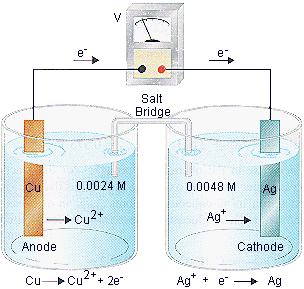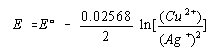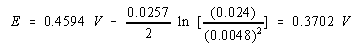Practice Problem 9

Calculate the potential at 25oC for the following cell.

 Cu | Cu2+(0.024 M) || Ag+(0.0048 M) | Ag

SolutionWe start by translating the line notation into an equation for the reaction.

Cu(s) + 2 Ag+(aq)Cu2+(aq) + 2 Ag(s)

We then look up the standard-state potentials for the two half-reactions and calculate the standard-state cell potential.

 Oxidation: CuCu2+ + 2 e- Eoox = -(0.3402 V) Reduction: Ag+ + e-Ag Eored = 0.7996 V ¯¯¯¯¯¯¯¯¯¯¯¯¯¯¯¯¯¯¯¯¯¯¯ Eo = Eored + Eoox = 0.4594 V

We now set up the Nernst equation for this cell, noting that n is 2 because two electrons are transferred in the balanced equation for the reaction.Substituting the concentrations of the Cu2+ and Ag+ ions into this equation gives the value for the cell potential.